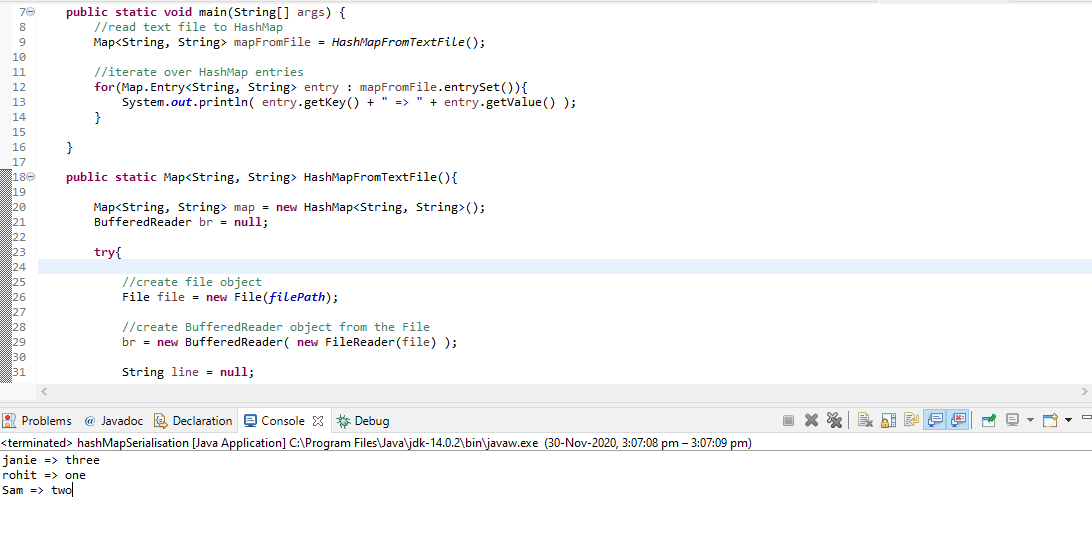Open in App
Not now

# Reading Text File into Java HashMap

• Last Updated : 28 Dec, 2020

We will look into the Method that allows us to read the HashMap Text from the file or how we can De-serialised the File

De-Serialization: Here we are reproducing the HashMap object and it’s content from a serialized file.

Approach:

Firstly the method/Function HashMapFromTextFile will have the Method bufferedReader which read the Line of the text File and insert into the map and then return the Map

`bf = new BufferedWriter( new FileWriter(file_name) );`
 `// Java program to reading``// text file to HashMap`` ` `import` `java.io.*;``import` `java.util.*;`` ` `class` `GFG {``    ``final` `static` `String filePath``        ``= ``"F:/Serialisation/write.txt"``;``    ``public` `static` `void` `main(String[] args)``    ``{`` ` `        ``// read text file to HashMap``        ``Map mapFromFile``            ``= HashMapFromTextFile();`` ` `        ``// iterate over HashMap entries``        ``for` `(Map.Entry entry :``             ``mapFromFile.entrySet()) {``            ``System.out.println(entry.getKey() + ``" : "``                               ``+ entry.getValue());``        ``}``    ``}`` ` `    ``public` `static` `Map HashMapFromTextFile()``    ``{`` ` `        ``Map map``            ``= ``new` `HashMap();``        ``BufferedReader br = ``null``;`` ` `        ``try` `{`` ` `            ``// create file object``            ``File file = ``new` `File(filePath);`` ` `            ``// create BufferedReader object from the File``            ``br = ``new` `BufferedReader(``new` `FileReader(file));`` ` `            ``String line = ``null``;`` ` `            ``// read file line by line``            ``while` `((line = br.readLine()) != ``null``) {`` ` `                ``// split the line by :``                ``String[] parts = line.split(``":"``);`` ` `                ``// first part is name, second is number``                ``String name = parts[``0``].trim();``                ``String number = parts[``1``].trim();`` ` `                ``// put name, number in HashMap if they are``                ``// not empty``                ``if` `(!name.equals(``""``) && !number.equals(``""``))``                    ``map.put(name, number);``            ``}``        ``}``        ``catch` `(Exception e) {``            ``e.printStackTrace();``        ``}``        ``finally` `{`` ` `            ``// Always close the BufferedReader``            ``if` `(br != ``null``) {``                ``try` `{``                    ``br.close();``                ``}``                ``catch` `(Exception e) {``                ``};``            ``}``        ``}`` ` `        ``return` `map;``    ``}``}`